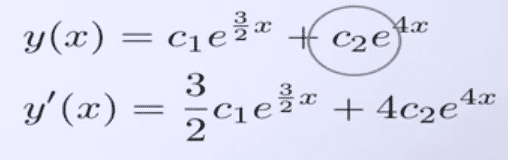# The integral of e^x

## Homework Statement

I thought sometimes the integral of e^x is xe^x. Under what circumstances is the integral of e^x = xe^x? I think it has something to do with u substitution.

Ray Vickson
Science Advisor
Homework Helper
Dearly Missed

## Homework Statement

I thought sometimes the integral of e^x is xe^x. Under what circumstances is the integral of e^x = xe^x? I think it has something to do with u substitution.

Under NO circumstances is the integral of e^x equal to x e^x. I cannot imagine why you think that would hold.

RGV

what about the derivative?

DryRun
Gold Member
The derivative of $e^x$ is: $$e^x .\frac{d(x)}{dx}$$

HallsofIvy
Science Advisor
Homework Helper
The derivative of $xe^x$ is, by the product rule $(x)'e^x+ (x)(e^x)'= 1(e^x)+ x(e^x)= xe^x+ e^x= (x+ 1)e^x$. As Ray Vickson said, the integral of $xe^x$ is NOT equal to itself and neither is the derivative.

The only functions having the property that their derivative is equal to the function itself is a constant times $e^x$.

DryRun
Gold Member
The derivative of $xe^x$ is, by the product rule $(x)'e^x+ (x)(e^x)'= 1(e^x)+ x(e^x)= xe^x+ e^x= (x+ 1)e^x$. As Ray Vickson said, the integral of $xe^x$ is NOT equal to itself and neither is the derivative.

The only functions having the property that their derivative is equal to the function itself is a constant times $e^x$.

I don't think anyone could have explained it better. This should resolve your confusion, robertjford80.

Here's an exampleWhat's going on here? It clear says that the derivative of

c1e(3/2)x[ = (3/2)c1e(3/2)x

SammyS
Staff Emeritus
Science Advisor
Homework Helper
Gold Member
Here's an exampleWhat's going on here? It clear says that the derivative of

c1e(3/2)x[ = (3/2)c1e(3/2)x
What's going on with the derivative of c1e(3/2)x[ is mainly the chain rule.

so the integral of e^2x is e^2x and the derivative of e^x is e^x but the derivative of e^2x is 2e^2x, is that right?

DryRun
Gold Member
so the integral of e^2x is e^2x and the derivative of e^x is e^x but the derivative of e^2x is 2e^2x, is that right?

Correct, except for the integral of ##e^{2x}## which is ##\frac{e^{2x}}{2}##

<deleted>

well, why don't you use the chain rule with e^x which would make it xe^x?

{this referred to number nine's deleted post} i saw it before he deleted it.

well, why don't you use the chain rule with e^x which would make it xe^x?

{this referred to number nine's deleted post} i saw it before he deleted it.

The chain rule is to multiply by the derivative, and the derivative of x is 1.

If it helps, d/dx (ex) = 1 * ex

thanks villyer, I hadn't thought about that.

Ray Vickson
Science Advisor
Homework Helper
Dearly Missed
so the integral of e^2x is e^2x and the derivative of e^x is e^x but the derivative of e^2x is 2e^2x, is that right?

No, the first statement is not right, and is not what you asked originally. The indefinite integral of exp(a*x) for constant a is (1/a)*exp(a*x) + C; the derivative of exp(a*x) is a*exp(a*x). When a = 1 these both give just exp(x). For a = 2 they give (1/2) exp(2x) and 2 exp(2x), respectively.

RGV

DryRun
Gold Member
thanks villyer, I hadn't thought about that.

The derivative of $e^x$ is: $$e^x .\frac{d(x)}{dx}$$

That's exactly what i said before.

if you're talking about post 4, then i don't think you provided enough info to convey that

DryRun
Gold Member
if you're talking about post 4, then i don't think you provided enough info to convey that
The derivative of $e^x$ is: $$e^x .\frac{d(x)}{dx}$$
It's obvious that $\frac{dx}{dx}=1$ which gives $e^x .1=e^x$. Unless, you didn't know that, but it's really a basic notion of the principles of differentiation.
You should go over the basic principles, as it should help you to understand ##e^x## and the others more complicated that will follow.

if it was obvious i would not have posted the question

if it was obvious i would not have posted the question

d(ex)/dx = ex

And so

∫ex dx = ___________ ....(i)

Note that integration is just reverse of differentiation.

If you want to verify this , then its simple ! Differentiate the left hand side of equation (i) with respect to x and see if its equal to ex. It will work.

If you want to prove it then analyze it by means of graph of f(x)=ex.

And note if you do this :

d(ex)/d(e) = xex-1

But it can never be xex !

Last edited: